PHY.K02UF Molecular and Solid State Physics

## Miller indices

### The translation vector

Every point of a Bravais lattice can be reached from the origin by a translation vector of the form,

$\begin{equation} \vec{T}_{hkl} = h\vec{a}_1 + k\vec{a}_2 + l\vec{a}_3, \end{equation}$

where $\vec{a}_1$, $\vec{a}_2$, $\vec{a}_3$ are the primitive lattice vectors and $h$, $k$, and $l$ are integers called the Miller indices. The direction that the vector $\vec{T}_{hkl}$ points is denoted with square brackets, $[hkl]$. If there are directions that are equivalent due to symmetry any of the equivalent directions are specified by angular brackets <$hkl$>.

For example, in a cubic system:
<100> = , , , [-100], [0-10], [00-1]
<110> = , [-1-10], [1-10], [-110], , [-10-1], [-101], [10-1], , [0-1-1], [0-11], [01-1].

Often the minus signs are drawn as overlines [-10-1] = $\left[\overline{1}0\overline{1}\right]$. This is pronounced one-bar zero one-bar.

### Planes

Using Miller indices, planes are denoted with curved brackets $(hkl)$. The $(hkl)$ plane intercepts the axis in the direction of $\vec{a}_1$ at $\frac{|\vec{a}_1|}{h}$, the axis in the direction of $\vec{a}_2$ at $\frac{|\vec{a}_2|}{k}$, and the axis in the direction of $\vec{a}_3$ at $\frac{|\vec{a}_3|}{l}$.

Equivalent planes are denoted with curly brackets $\{hkl\}$. Three points that can be used to define the $(hkl)$ plane are $\left( \frac{a_{1x}}{h},\frac{a_{1y}}{h},\frac{a_{1z}}{h} \right)$, $\left(\frac{a_{2x}}{k},\frac{a_{2y}}{k},\frac{a_{2z}}{k} \right)$, and $\left(\frac{a_{3x}}{l},\frac{a_{3y}}{l},\frac{a_{3z}}{l}\right)$. The normal vector to this plane can be determined by taking the cross product of two vectors in the plane. If $(h,k,l \ne 0)$, two suitable vectors are $\vec{v}_1=\frac{\vec{a}_1}{h}-\frac{\vec{a}_3}{l}$ and $\vec{v}_2=\frac{\vec{a}_2}{k}-\frac{\vec{a}_3}{l}$. The unit vector normal to the $(hkl)$ plane is,

$$\hat{n}_{hkl}=\frac{\vec{v}_1\times \vec{v}_2}{|\vec{v}_1\times \vec{v}_2|}.$$

For cubic crystals, $\vec{T}_{hkl}$ is normal to $(hkl)$ and parallel to $\hat{n}_{hkl}$ but this is not generally true for crystals with other symmetries.

Primitive lattice vectors:

 $\vec{a}_1=$ $\hat{x}+$ $\hat{y}+$ $\hat{z}$ [m] $\vec{a}_2=$ $\hat{x}+$ $\hat{y}+$ $\hat{z}$ [m] $\vec{a}_3=$ $\hat{x}+$ $\hat{y}+$ $\hat{z}$ [m]

Miller indices:

 $h=$ $k=$ $l=$

Primitive unit cells:

Conventional unit cells:

JSmol can be used to draw crystalographic planes of different crystal structures. The JSmol models of some common crystal structures are linked below.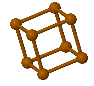Simple Cubic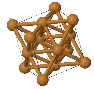Face Centered Cubic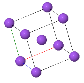Body Centered Cubic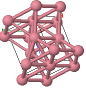Hexagonal Close Packed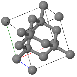Diamond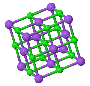NaCl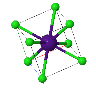CsCl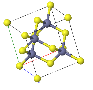ZincblendeWurzite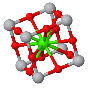Perovskite